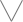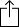C
D
E
F
G
A
B
Chord
Scale
Custom
3nps
CAGED
None
Notes
Degrees
Intervals
None

1
2
3
4
5
6
7
1
2
3
4
5
6
7
8
9
10
11
12
13
14
15
16
17
18
19
20
21
22
23
24
E
B
G
D
A
E
B
F
F
G
A
A
B
B
C
D
D
E
E
F
F
G
A
A
B
B
C
D
D
E
E
C
D
D
E
E
F
F
G
A
A
B
B
C
D
D
E
E
F
F
G
A
A
B
B
A
A
B
B
C
D
D
E
E
F
F
G
A
A
B
B
C
D
D
E
E
F
F
G
E
E
F
F
G
A
A
B
B
C
D
D
E
E
F
F
G
A
A
B
B
C
D
D
B
B
C
D
D
E
E
F
F
G
A
A
B
B
C
D
D
E
E
F
F
G
A
A
F
F
G
A
A
B
B
C
D
D
E
E
F
F
G
A
A
B
B
C
D
D
E
E
C
D
D
E
E
F
F
G
A
A
B
B
C
D
D
E
E
F
F
G
A
A
B
B

B Minor for 7 String Guitar - 3nps Chart

A B Minor chord, also written as Bm, B- or Bmin contains the notes B, D and F#. The chord is comprised of the intervals "m3" and "M3".

B
D
Fm3
M3
Play scaleand then